# Class 6 Maths NCERT Solutions for Chapter 3 Playing with Numbers Ex 3.7

## Playing with Numbers

Question 1.
Renu purchases two bags of fertilizer of weights 75 kg and 69 kg. Find the maximum value of weight which can measure the weight of the fertilizer e×act number of times.

Solution :
Factors of 75 are 1, 3, 5, 15, 25 and 75.
Factors of 69 are 1, 3, 23 and 69.
∴ Common factors of 75 and 69 are 1 and 3.
Highest of these common factors is 3.
∴ H.C.F. of 75 and 69 is 3.
Hence, the maximum value of weight which can measure the weight of the fertilizer e×act number of times is 3 kg.

Question 2.
Three boys step off together from the same spot. Their steps measure 63 cm, 70 cm, and 77 cm, respectively. What is the minimum distance each should cover so that all can cover the distance in complete steps?

Solution :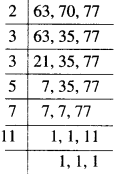∴ L.C.M. of 63, 70 and 77
= 2 × 3 × 3 × 5 × 7 × 11 = 6930.
Hence, the minimum distance each should cover so that all can cover the distance in complete steps is 6930 cm.

Question 3.
The length, breadth, and height of a room are 825 cm, 675 cm, and 450 cm, respectively. Find the longest tape which can measure the three dimensions of the room exactly.

Solution :
Factors of 825 are 1, 3, 5, 11, 15, 25, 33, 55,75,165, 275 and 825.
Factors of 675 are 1, 3, 5,9,15, 25, 27,45,75, 135, 225 and 675.
Factors of 450 are 1,2,3,5,6,9,10,15,18,25, 30,45, 50, 75, 90, 150, 225 and 450.
∴ Common factors of 825, 675 and 450 are 1,3,5,15, 25 and 75.
Highest of these common factors is 75.
Hence, the length of the longest tape which can measure the three dimensions of the room exactly is 75 cm.

Question 4.
Determine the smallest 3-digit number which is exactly divisible by 6, 8 and 12.

Solution :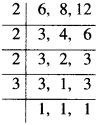∴ L.C.M. of 6, 8 and 12 = 2 × 2 × 2 × 3 = 24. Multiples of 24 are 24,48,72,96,120,144,
Hence, the smallest 3-digit number which is exactly divisible by 6, 8 and 12 is 120.

Question 5.
Determine the largest 3-digit number exactly divisible by 8, 10 and 12.

Solution :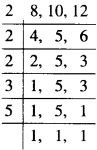∴ L.C.M. of 8,10 and 12 = 2×2×2×3×5
= 120.
Multiples of 120 are :
120 × 1 = 120,120 × 2 = 240,120 × 3 = 360,120 × 4 = 480,120 × 5 = 600,120 × 6 = 720,120 × 7 = 840,
120 × 8 = 960,120 × 9 = 1080,
Hence, the largest 3-digit number exactly divisible by 8, 10 and 12 is 960.

Question 6.
The traffic lights at three different road crossings change after every 48 seconds, 72 seconds and 108 seconds respectively. If they change simultaneously at 7 am at what time will they change simultaneously again?

Solution :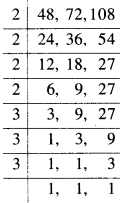∴ L.C.M. of 48,72 and 108 = 2 × 2 × 2 × 2 × 3 × 3 × 3 = 432.
432 seconds = 7 min 12 seconds.
Hence, they will change simultaneously again after 7 min 12 seconds from 7 a.m.

Question 7.
Three tankers contain 403 liters, 434 liters and 465 liters of diesel respectively. Find the ma×imum capacity of a container that can measure the diesel of the three containers e×act a number of times.

Solution :
Factors of 403 are 1, 13, 31 and 403. Factors of 434 are 1, 2, 7, 14, 31, 62, 217 and 434.
Factors of 465 are 1, 3, 5, 15, 31, 93, 155 and 465.
Common factors of 403,434 and 465 are 1 and 31.
Highest of these common factors is 31.
∴ H.C.F. of 403. 434 and 465 is 31.
Hence, the maximum capacity of the container that can measure the diesel of the three containers an e×act number of times is 31 litres.

Question 8.
Find the least number which when divided by 6, 15 and 18 leave remainder 5 in each case.

Solution :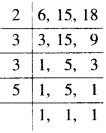∴ L.C.M. of 6, 15 and 18 = 2 × 3 × 3 × 5 = 90.
Hence, the required number is 90 + 5 i.e., 95.

Question 9.
Find the smallest four digit number which is divisible by 18, 24 and 32.

Solution :∴ L.C.M. = 2 × 2 × 2 × 2 × 2 × 3 × 3 = 288.
Multiples of 288 are :
288 × 1 = 288, 288 × 2 = 576, 288 × 3 = 864, 288×4= 1152,
Hence, the smallest four digit number which is divisible by 18, 24 and 32 is 1152.

Question 10.
Find the L.C.M. of the following numbers:

(a) 9 and 4
(b) 12 and 5
(c) 6 and 5
(d) 15 and 4.
Observe a common property in the obtained L.C.M.s. Is L.C.M. the product of two numbers in each case?

Solution :
(a)
9 and 4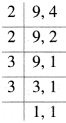∴ L.C.M. of 9 and 4 = 2 × 2 × 3 × 3 = 36 (= 9 × 4).

(b)
12 and 5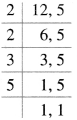∴ L.C.M. of 12 and 5 = 2×2×3×5 = 60 (= 12 × 5).

(c)
6 and 5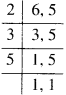∴ L.C.M. of 6 and 5 = 2×3×5 = 30 (= 6 × 5).

(d)
15 and 4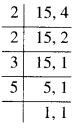∴ L.C.M. of 15 and 4 = 2 × 2 × 3 × 5 = 60 (=15×4).
We observe a common property in the obtained L.C.M.’s that L.C.M. is the product of two numbers in each case.

Question 11.
Find the L.C.M. of the following numbers in which one number is the factor of the other.

(a) 5, 20
(b) 6, 18
(c) 12, 48
(d) 9, 45.
What do you observe in the results obtained?

Solution :
(a)
5, 20
Prime factorisations of 5 and 20 are as follows: 5 = 5
20 = 2 × 2 × 5 ∴ L.C.M. of 5 and 20
=2×2×5 = 20.

(b)
6, 18
Prime factorisations of 6 and 18 are as follows:
6 = 2×3 18 = 2×3×3
∴ L.C.M. of 6 and 18 = 2×3×3 = 18.

(c)
12, 48
Prime factorisations of 12 and 48 are as follows:
12 = 2 × 2 × 3
48 = 2×2×2×2×3
∴ L.C.M. of 12 and 48 = 2 × 2 × 2 × 2 × 3 = 48.

(d)
9, 45
Prime factorisations of 9 and 45 are as follows:
9 = 3×3
45 = 3 × 3 × 5
∴ L.C.M. of 9 and 45 = 3 × 3 × 5 = 45.
In the results obtained, we observe that L.C.M. of the two numbers in which one number is the factor of the other is the greater number.